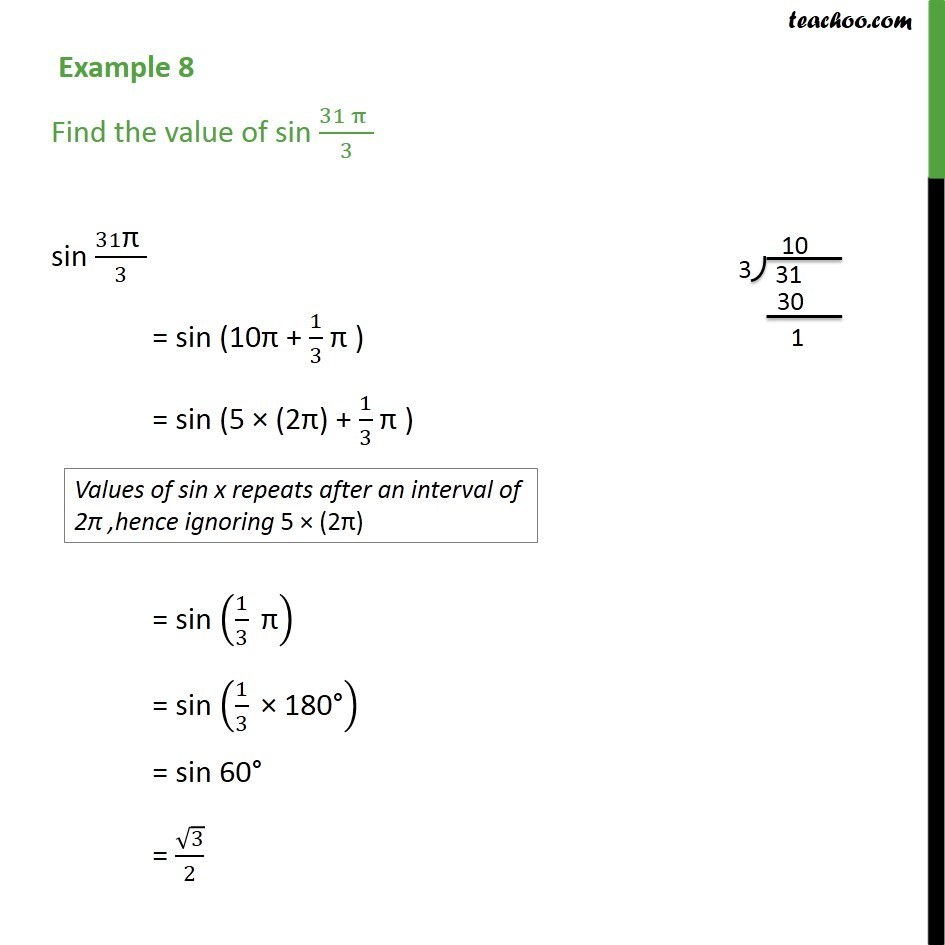Learn All Concepts of Chapter 2 Class 11 Relations and Function - FREE. Check - Trigonometry Class 11 - All Concepts1. Chapter 3 Class 11 Trigonometric Functions
2. Concept wise
3. Finding Value of trignometric functions, given angle

Transcript

Example 8 Find the value of sin (31" " π" " )/3 sin 31"π " /3 = sin (10π + 1/3 "π ") = sin (5 × (2π) + 1/3 "π ") = sin (1/3 " π" ) = sin (1/3 " × 180°" ) = sin 60° = √3/2

Finding Value of trignometric functions, given angle

About the AuthorDavneet Singh
Davneet Singh is a graduate from Indian Institute of Technology, Kanpur. He has been teaching from the past 9 years. He provides courses for Maths and Science at Teachoo.